International
Tables for
Crystallography
Volume B
Reciprocal space
Edited by U. Shmueli

International Tables for Crystallography (2010). Vol. B, ch. 2.5, pp. 371-372   | 1 | 2 |

## Section 2.5.6.5. Filtered backprojection

B. K. Vainshteinc and P. A. Penczekg

#### 2.5.6.5. Filtered backprojection

| top | pdf |

The method of filtered backprojection (FBP) is based on inversion formulae (2.5.6.11)(in two dimensions) or (2.5.6.14)(in three dimensions). It comprises the following steps: (i) a Fourier transform of each projection is computed; (ii) Fourier transforms of projections are multiplied by filters that account for a particular distribution of projections in Fourier space; (iii) the filtered projections are inversely Fourier transformed; (iv) real-space backprojection of processed projections yields the reconstruction. The method is particularly attractive due to the fact that the reconstruction calculated using simple real-space backprojection can be made efficient if the filter function is easy to compute.

In two dimensions with uniformly distributed projections the weighting function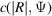in Fourier space is the ramp function'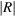[(2.5.6.13)]. In two dimensions with nonuniformly distributed projections, when the analytical form of the distribution of projections is not known, an appropriate approximation tohas to be found. A good choice is to select weights such that the backprojection integral becomes approximated by a Riemann sum (Penczek et al., 1996),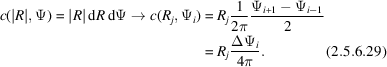For a given set of angles the weights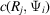are easily computed (Fig. 2.5.6.5).Figure 2.5.6.5 | top | pdf |Nonuniform distribution of projections. The projection weights for the reconstruction algorithms are chosen such that the backprojection integral becomes approximated by a Riemann sum and are equal to the angular length of an arc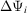(2.5.6.29). In Fourier space, projections of an object with real-space radius D form rectangles with width 1/D. In the exact filter backprojection reconstruction method, the weights are derived based on the amount the overlap between projections (2.5.6.29).

In three dimensions, the weighting (2.5.6.29)is applicable in a single-axis tilt data-collection geometry, where the 3D reconstruction can be calculated as a series of independent 2D reconstructions. In the general 3D case, the analogue of weighting (2.5.6.29)cannot be used, as the data are given in the form of 2D projections and it is not immediately apparent what fraction of the 3D Fourier volume is occupied by Fourier pixels in projections. However, the analogue of weighting (2.5.6.29)can be used in the inversion of 3D Radon transforms or in a direction inversion of 3D ray transforms that is based on an intermediate step of converting 2D projection data to 1D projection data, as described in Section 2.5.6.6.

In order to arrive at a workable solution, the weighting functions applicable to 2D projections are constructed based on an explicitly or implicitly formulated concept of the local density' of projections. This concept was introduced by Bracewell (Bracewell & Riddle, 1967), who suggested for a 2D case of non­uniformly distributed projections a heuristic weighting function,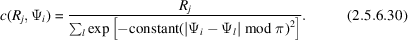The weighting function (2.5.6.30)can be easily extended to three dimensions; however, it has a major disadvantage that for a uniform distribution of projections it does not approximate well the weighting function (2.5.6.29), which we consider optimal.

Radermacher et al. (1986) proposed a derivation of a general weighting function using a deconvolution kernel calculated for a given (nonuniform) distribution of projections and, in modification of (2.5.6.14), a finite length of backprojection (Fig. 2.5.6.3). Such a truncated' backprojection is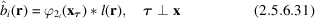with projection directions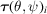and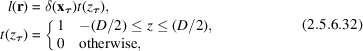where D is the diameter of the object or the length of the backprojection l. By taking the Fourier transform of (2.5.6.31)and using the central section theorem (2.5.6.8), we obtain a 3D Fourier transform of the backprojected projection,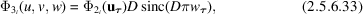and the 3D reconstruction is obtained by the inverse Fourier transform of the sum of contributions given by (2.5.6.33),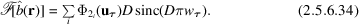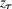and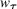are variables in real and Fourier spaces, respectively, both extending in the direction of the projection direction.

In this analysis, the transfer function of the backprojection algorithm is obtained by setting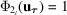, that is by finding the response of the algorithm to the input composed of delta functions in real space. This yields the inversion formula for a general weighted backprojection algorithm: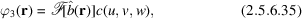with the weighting function given by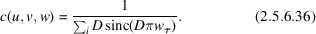The general weighting function (2.5.6.36)is consistent with analytical solutions (2.5.6.11)and (2.5.6.14), as it can be shown that by assuming infinite support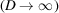and continuous and uniform distribution of projection directions, in two dimensions one obtains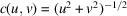(Radermacher, 2000).

The derivation of (2.5.6.36)is based on the analysis of continuous functions and its direct application to discrete data results in reconstruction artifacts; therefore, Radermacher (1992) proposed attenuating the sinc functions in (2.5.6.36)by exponent functions with decay depending on the diameter of the structure D, or simply replacing the sinc functions by exponent functions. This, however, reduces the concept of the weighting function corresponding to the deconvolution to the concept of the weighting function representing the local density' of projections (2.5.6.30). The general weighted backprojection reconstruction is implemented in SPIDER (using exponent-based weighting functions) (Frank et al., 1996), Xmipp (Sorzano et al., 2004) and SPARX (Hohn et al., 2007).

Harauz & van Heel (1986) proposed basing the calculation of the density of projections, thus the weighting function, on the overlap of Fourier transforms of projections in Fourier space. Although the concept is general, it can be easily approached in two dimensions. If the diameter of the object is D, the width of a Fourier transform of a projection is 2/D (Fig. 2.5.6.5), as follows from the central section theorem (2.5.6.8). Harauz and van Heel postulated that the weighting should be inversely proportional to the sum of geometrical overlaps between a given central section and the remaining central sections. For a pair of projections il, this overlap is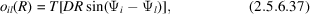where T represents the triangle function (selected by the authors because it can be calculated efficiently). Also, owing to the Friedel symmetry of central sections, the angles in (2.5.6.37)are restricted such that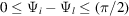. In this formulation, the overlap is limited to the maximum frequency,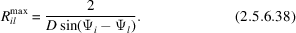Thus, the overlap function becomes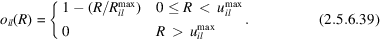In effect, the weighting function, called by the authors an `exact filter', is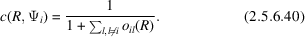The weighting function (2.5.6.40)easily extends to three dimensions; however, the calculation of the overlap between central sections in three dimensions (represented by slabs) is more elaborate (Harauz & van Heel, 1986). The method is conceptually simple and computationally efficient. However, (2.5.6.40)does not approximate the correct weighting well for a uniform distribution of projections [i.e., it should yield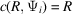]. This, as can be seen by integrating (2.5.6.39)over the whole angular range, is not the case. The exact filter backprojection reconstruction is implemented in the IMAGIC (van Heel et al., 1996), SPIDER (Frank et al., 1996) and SPARX (Hohn et al., 2007) packages.

The 3D reconstruction methods based on filtered backprojection are commonly used in single-particle reconstruction. The reasons are: their versatility, ease of implementation, and – in comparison with iterative methods – good computational efficiency. Unlike in iterative methods, there are no parameters to adjust, although it has been noted that the results depend on the value of the diameter D of the structure in all three weighting functions [(2.5.6.30), (2.5.6.36)and (2.5.6.38)], so the performance of the reconstruction algorithm can be optimized for a particular data-collection geometry by changing the value of D (Paul et al., 2004). However, because computation of the weighting function involves calculation of pairwise distances between projections, the computational complexity is proportional to the square of the number of projections and for large data sets these methods become inefficient. It also has to be noted that the weighting functions (2.5.6.30), (2.5.6.36)and (2.5.6.40)remain approximations of the correct weighting function (2.5.6.29).

### References

Bracewell, R. N. & Riddle, A. C. (1967) Inversion of fan-beam scans in radio astronomy. Astrophys. J. 150, 427–434.
Frank, J., Radermacher, M., Penczek, P., Zhu, J., Li, Y., Ladjadj, M. & Leith, A. (1996). SPIDER and WEB: processing and visualization of images in 3D electron microscopy and related fields. J. Struct. Biol. 116, 190–199.
Harauz, G. & van Heel, M. (1986). Exact filters for general geometry three-dimensional reconstruction. Optik, 73, 146–156.
Heel, M. van, Harauz, G. & Orlova, E. V. (1996). A new generation of the IMAGIC image processing system. J. Struct. Biol. 116, 17–24.
Hohn, M., Tang, G., Goodyear, G., Baldwin, P. R., Huang, Z., Penczek, P. A., Yang, C., Glaeser, R. M., Adams, P. D. & Ludtke, S. J. (2007). SPARX, a new environment for cryo-EM image processing. J. Struct. Biol. 157, 47–55.
Paul, D., Patwardhan, A., Squire, J. M. & Morris, E. P. (2004) Single particle analysis of filamentous and highly elongated macromolecular assemblies. J. Struct. Biol. 148, 236–250.
Penczek, P. A., Zhu, J. & Frank, J. (1996). A common-lines based method for determining orientations for N > 3 particle projections simultaneously. Ultramicroscopy, 63, 205–218.
Radermacher, M. (1992). Weighted back-projection methods. In Electron Tomography, edited by J. Frank, pp. 91–115. New York: Plenum.
Radermacher, M. (2000). Three-dimensional reconstruction of single particles in electron microscopy. In Image Analysis: Methods and Applications, edited by D.-P. Häder, pp. 295–328. Boca Raton: CRC Press.
Radermacher, M., Wagenknecht, T., Verschoor, A. & Frank, J. (1986). A new 3-D reconstruction scheme applied to the 50S ribosomal subunit of E. coli. J. Microsc. 141, RP1–RP2.
Sorzano, C. O. S., Marabini, R., Velazquez-Muriel, J., Bilbao-Castro, J. R., Scheres, S. H. W., Carazo, J. M. & Pascual-Montano, A. (2004). XMIPP: a new generation of an open-source image processing package for electron microscopy. J. Struct. Biol. 148, 194–204.